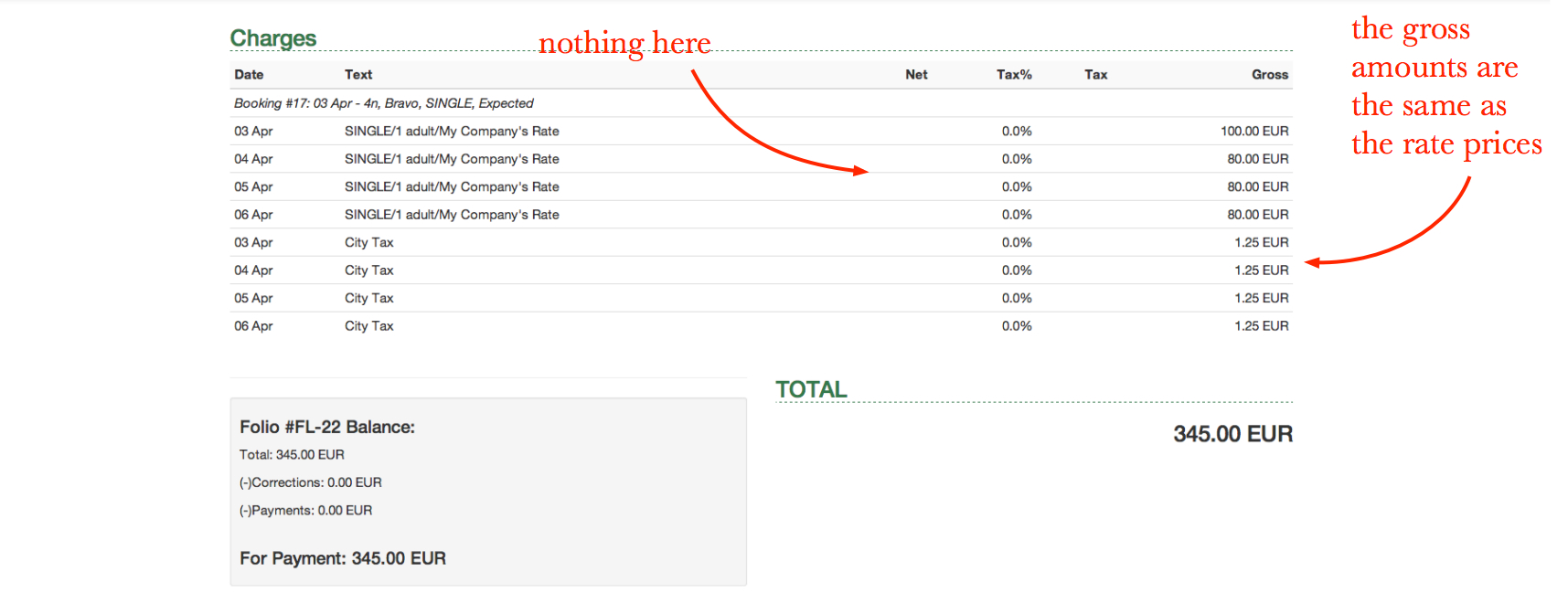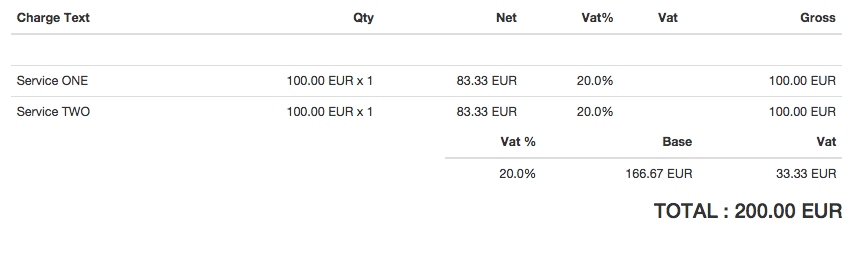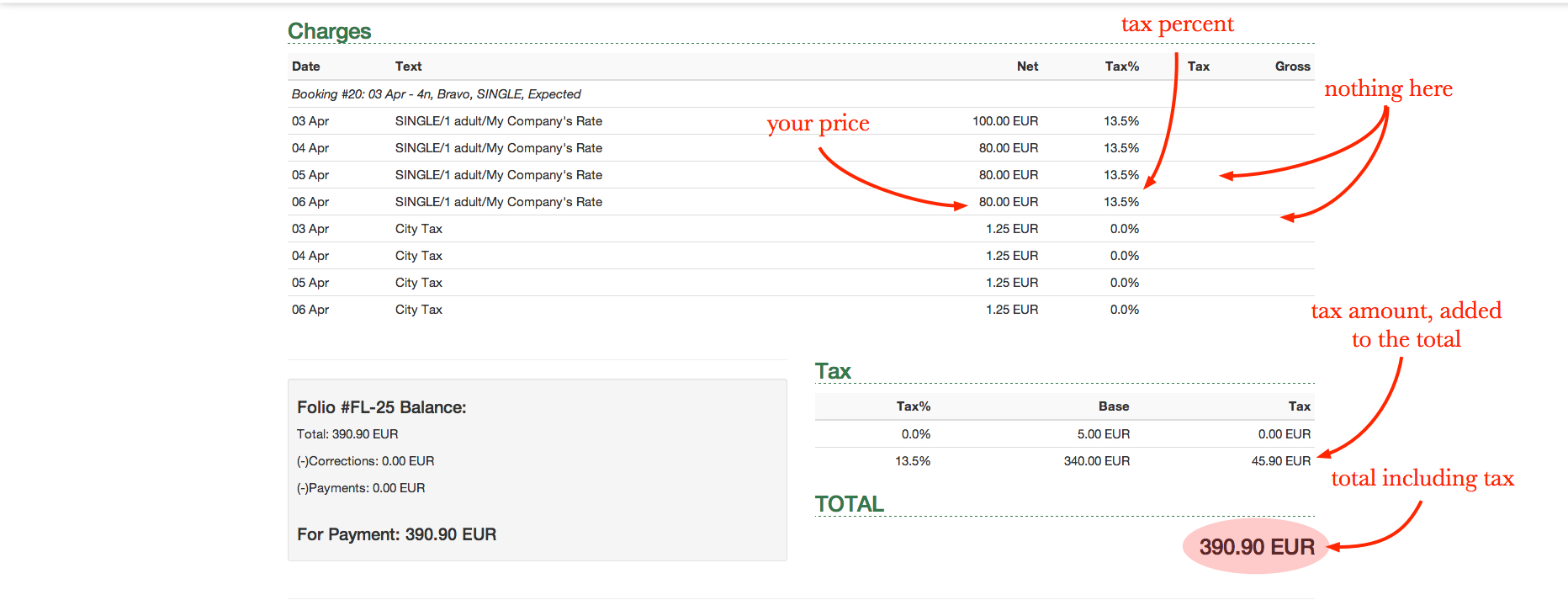The tax settings determine how the information in your invoices will be arranged and how the tax will be calculated.

Clock PMS offers its users a wide range of tax modes to be used:

• No tax
• Tax included in prices, rounding - per line
• Tax included in prices, rounding - total
• Tax not included in prices, rounding - per line
• Tax not included in prices, rounding – total

# Please note that the different rounding options may result in differences in the invoice total. And the values of the tax rates in Clock PMS can have up to 4 decimal places.

## 'No tax'• In the No Tax mode, the Net and Tax columns are empty, the Tax% column shows 0.0%, and the gross amounts are the same as the rate prices, because no tax is added to the rate prices.
• The charge and folio reports do not show net amounts and tax amounts.

## 'Tax included in prices, rounding - per line'1. For each folio line, the TAX BASE is calculated as per the following formula: Total/( 1 + Tax%/100). The resultingamount is rounded to the smallest monetary unit (usually 0.01):
• Example for 20% Tax and final price of 100 EUR: 100/1.2 = 83.33333…, the rounded amount is equal to 83.33.
2. For each folio line, the TAX is calculated as PRICETAX BASE = TAX:
• Example for 20% Tax: 100 - 83.33 = 16.67.
3. In the folio total section, the respective amounts are calculated by summing the lines:
• Example for 20% Tax: Two lines, each containing a final price of 100 EUR:

TOTAL TAX BASE = 83.33 + 83.33 = 166.66.

TOTAL TAX = 16.67 + 16.67 = 33.34

4. Note: Tax mode specifics: If you calculate the tax on the basis of the TOTAL amounts, you will not have the same result. Example for 20% Tax: 200/1.2 = 166.66666…, the rounded amount is equal to 166.67. And the resulting tax base amount as per this tax mode is 166.66. See also the other tax mode: 'Tax included in prices, rounding - total'

### Tax included in prices, rounding - total• The folio TOTAL is calculated by summing the gross prices of each line:
• Example: Two lines, each containing a gross price of 100 EUR, and their total is: 100 + 100 = 200 EUR
• On the basis of this TOTAL, the TAX BASE is calculated using the following formula: Total/( 1 + Tax%/100). The resulting amount is rounded to the smallest monetary unit (usually 0.01):
• Example for 20% Tax: 200/1.2 = 166.66666…, the rounded amount is 166.67
• The TAX amount is calculated as TOTALTAX BASE = TAX AMOUNT:
• Example for 20% Tax: 200 - 166.67 = 33.33. Thus calculated , the TAX BASE and TAX are presented in the folio total section.
• Additionally, the tax bases of each line are also calculated, as these amounts are rounded in each line:
• Example for 20% Tax: the tax base for each of two lines, containing a final price of 100 EUR, is calculated the following way: Tax base = 100 / 1.2 = 83.3333… = 83.33
Note: Tax mode specifics: The sum of the individual tax base amounts of the folio lines DOES NOT correspond to the folio tax base. Example for 20% Tax: The tax base of the whole folio, calculated as per the above formula: Total/( 1 + Tax%/100), is 166.67, and the sum of the tax bases of the individual folio lines is 83.33 + 83.33 = 166.66. See also the other tax mode: 'Tax included in prices, rounding - per line'

### 'Tax not included in prices, rounding - per line'• The tax amount of each line (charge) is calculated on the basis of the following formula:

Tax Value = Bankers' Rounding (Net Value * (Tax Rate / 100))

• The gross value for each line (charge) is equal to the sum of the net amount and the tax amount in this line.
• The gross amount of the invoice (invoice total) is equal to the sum of the values in the lines of the tax column.
• The total tax amount of the invoice is equal to the sum of the values in the lines of the tax column. For each tax rate, the sum of the corresponding tax amounts is shown in the reports.
• In the charge and folio reports, the net, tax and gross amounts are presented.

### 'Tax not included in prices, rounding – total'• No gross value for each line is calculated.
• The total net amount of the invoice is the sum of the net amounts in each line of the Net column.
• The total tax amount of the invoice is calculated on the basis of the following formula:

Rounding (Total Net Value * (Tax Rate / 100)). For each tax rate, the sum of the corresponding tax amounts is shown in the reports

• The total gross amount is calculated as per the following formula:

Total Gross Amount = Total Net Amount + Total Tax Amount.

• In the folio reports, there is information regarding the net, tax and gross amounts for each folio.
• In the charge reports, only the net values are presented, because the tax amounts are calculated on the basis of the folio totals instead of the sum of the tax amounts related to the individual charges. Therefore, this method is appropriate for companies following a document based revenue recognition principle.
Note: The round-half-to-even rounding method (Bankers' Rounding) is used in the above three cases.

### Tax Name and Default Tax Percent

• 'Tax name' - enter a short name for the tax. For example: VAT, TVA, MWSt, Moms, GST, Tax, etc.
• 'Default tax percent' - enter the most common tax value. It will be suggested automatically for new charges, new charge templates and new rates. If necessary, it can be changed for each specific instance.

### Different Tax Rates for Different Charges

Despite the option for a default tax rate, you can use different custom tax rates with each charge template, individual charge, rate. The same applies to city tax. Thus the tax rate for room charges can be 12%, and the tax rate for extra services – 20%.

Here is how you can set a custom tax rate:

#### Charge Templates

• Go to 'Settings' – 'Charge Template' and select a charge template to be edited
• In the applicable tax % field, enter the necessary value of the tax rate
• Click 'Save'
Note: Similarly, before posting a manual charge, enter a custom value of the tax rate in the applicable tax % field

#### Rates

• Go to 'Settings' – 'Rate Plan' and select a rate plan
• On the rate plan screen, click the Edit button
• In the applicable tax % field, enter the necessary value of the tax rate
• Click 'Save'

#### City Taxes

• Go to 'Settings' – 'City Tax'
• In the applicable tax % field, enter the necessary value of the tax rate
• Click 'Save'

City taxes are local fees, e.g. Resort Tax. They are collected from guests by being added to the room rates charged. For more info on how these city taxes are set and the possible city tax modes, see City Tax.

City taxes differ from actual taxes. The former are posted as charges on guests' folios and then these charges appear in revenue reports. City taxes are not calculated as a percentage of the respective room rates, but are added as absolute amounts to these room rates. The city taxes themselves can be taxed. In contrast to them, actual taxes are calculated as a percentage of the respective charges.

### Multiple Taxes

In the cases when several taxes apply to your sales, e.g. State Sale Tax and Local Tax, you can apply a combined tax rate. Use the 'Tax code' field to provide greater clarity and accountability. Here is an example:

• State Sale Tax Rate: 4%
• Local Rate: 4.875%
• Combined Rates:8.875%

Set the tax rate at 8.875 in the rate and enter “St.4% + Loc.4.875%” in the Tax code field.

### Surcharge

You can set a surcharge fee to apply to folios in Clock PMS. The surcharge is defined as a percent and is automatically calculated on the basis of the folio value. With the change of the folio value – the surcharge is also recalculated automatically.

The basis for the calculation of a surcharge is the value of the charges posted. Depending on the tax mode selected and the respective surcharge basis (the value of the charges), it may or may not include tax.

#### Setup

• Open 'Others' - 'Settings' - 'Tax Settings'
• In the 'Surcharge' section, fill in:
• Surcharge text (as you want it to appear on the folio)
• Surcharge percent
• Surcharge tax percent (tax percent to be levied on the surcharge)
• Save.

#### Surcharge percent for a specific folio

To change the surcharge percent for a given folio or practically remove it (setting a zero value):

• Open the folio
• Click the 'Currency/Name' button and fill in the new value of the surcharge.

In the charge reports, the surcharge turnovers are listed in the surcharge revenue group.